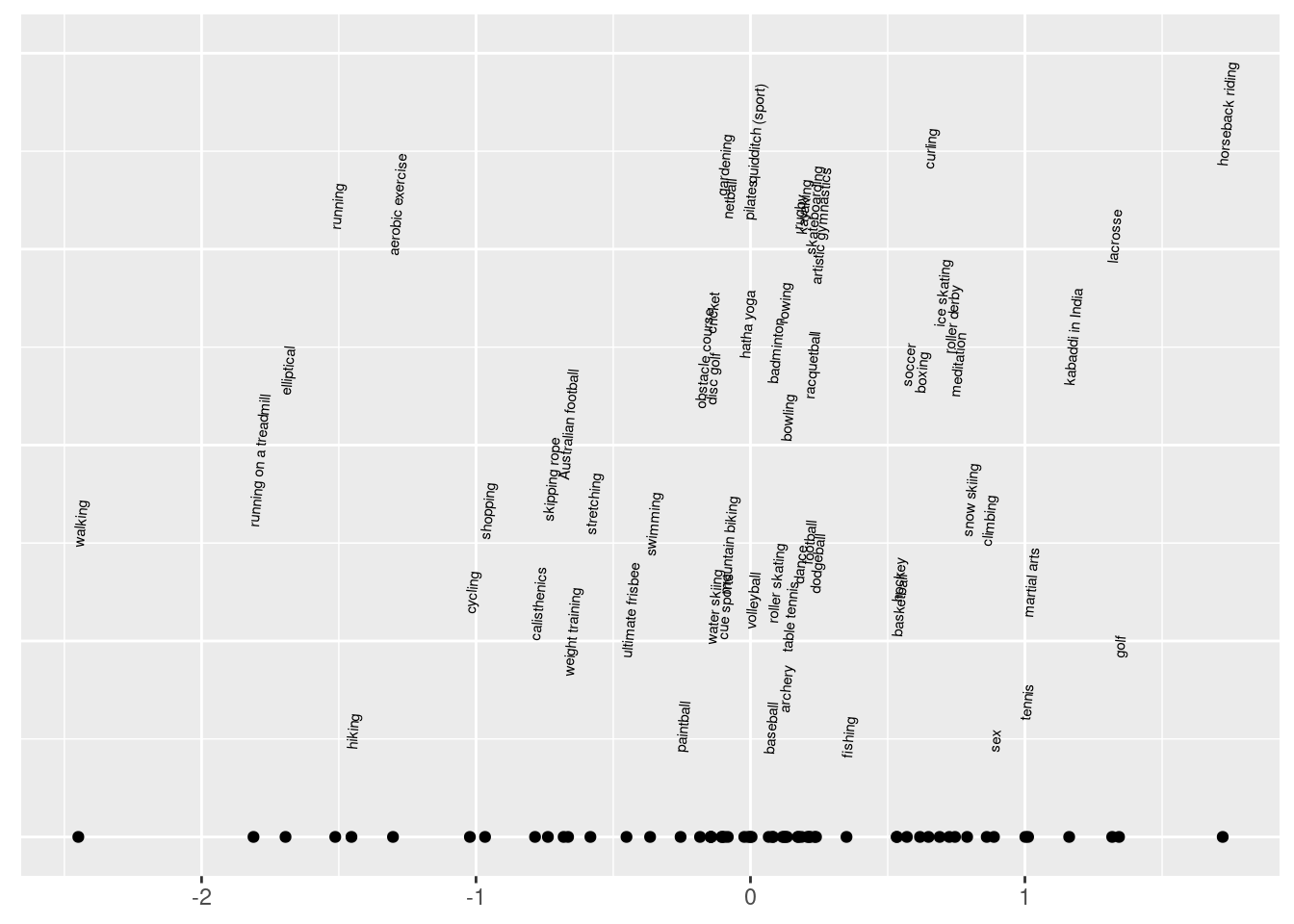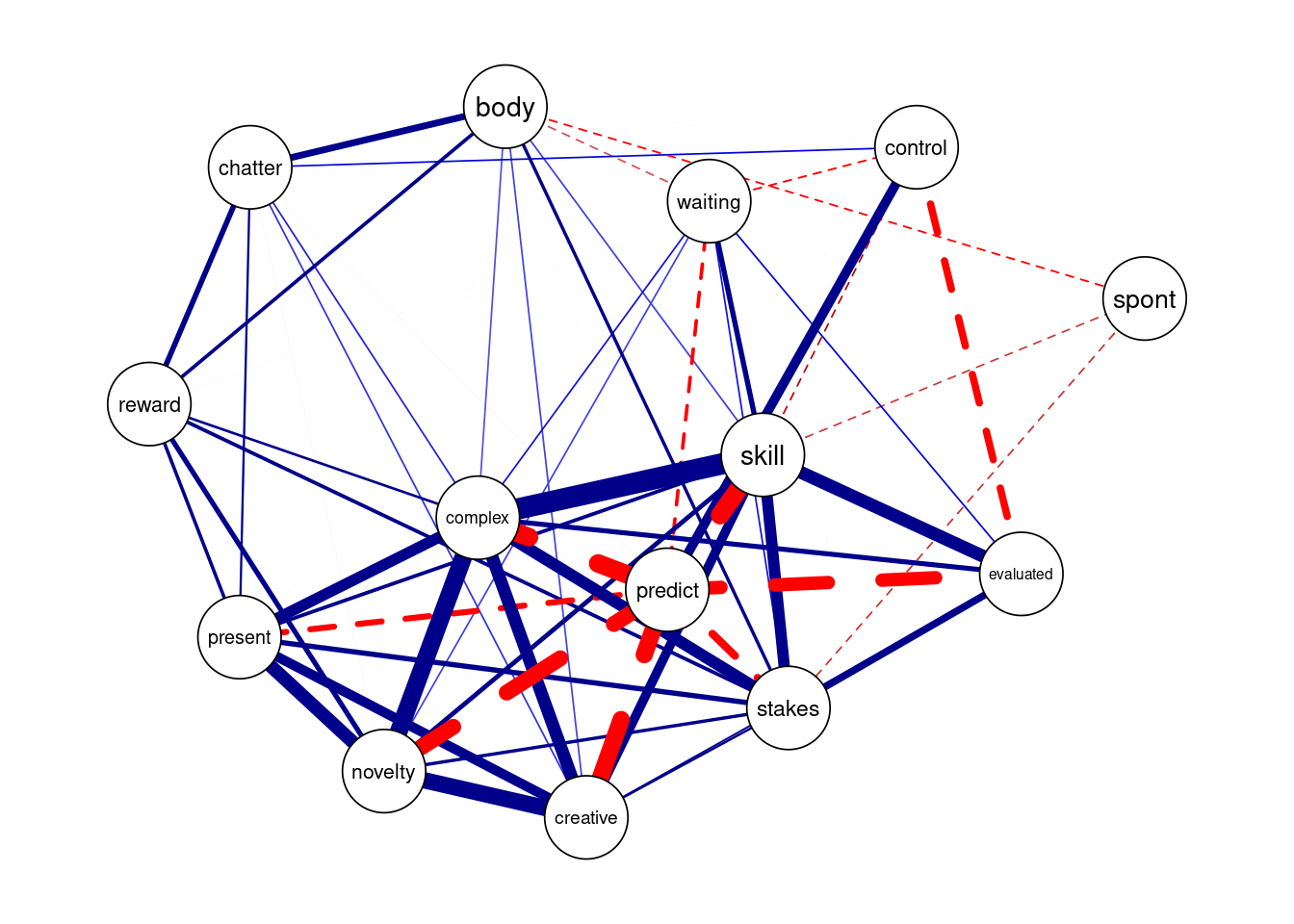# Overview

The pcFactorStan package for R provides convenience functions and pre-programmed Stan models related to analysis of paired comparison data. Its purpose is to make fitting models using Stan easy and easy to understand. pcFactorStan relies on the rstan package, which should be installed first. See here for instructions on installing rstan.

One situation where a factor model might be useful is when there are people that play in tournaments of more than one game. For example, the computer player AlphaZero (Silver et al. 2018) has trained to play chess, shogi, and Go. We can take the tournament match outcome data for each of these games and find rankings among the players. We may also suspect that there is a latent board game skill that accounts for some proportion of the variance in the per-board game rankings. This proportion can be recovered by the factor model.

Our goal may be to fit a factor model, but it is necessary to build up the model step-by-step. There are essentially three models: ‘unidim’, ‘correlation’, and ‘factor’. ‘unidim’ analyzes a single item. ‘correlation’ is suitable for two or more items. Once you have vetted your items with the ‘unidim’ and ‘correlation’ models, then you can try the ‘factor’ model. There is also a special model ‘unidim_adapt’. Except for this model, the other models require scaling constants. To find appropriate scaling constants, we will fit ‘unidim_adapt’ to each item separately.

# Brief tutorial

## Physical activity flow propensity

The R code below first loads rstan and pcFactorStan. We load loo for extra diagnostics, and qgraph and ggplot2 for visualization.

``````library(rstan)
library(pcFactorStan)
library(loo)
library(qgraph)
library(ggplot2)
library(Matrix)``````

Next we take a peek at the data.

``head(phyActFlowPropensity)``
pa1 pa2 skill predict novelty creative complex goal1 feedback1 chatter waiting body control present spont stakes evaluated reward
mountain biking tennis 1 -1 -2 1 1 1 1 -2 1 1 1 1 1 1 2 0
mountain biking tennis 1 2 -1 -1 -1 0 2 1 2 0 1 0 0 1 2 -1
ice skating running -2 1 -1 -2 -1 1 1 -2 -2 -1 0 0 -1 -1 -1 0
climbing rowing -2 2 -2 -2 -2 0 -1 -1 -1 -1 -1 -1 1 0 0 0
card game gardening 0 0 0 0 2 0 0 0 -2 2 1 0 0 2 -2 2
dance table tennis 0 -2 -1 -1 0 -1 -1 -1 0 0 0 0 0 0 0 1

These data consist of paired comparisons of 87 physical activities on 16 flow-related facets. Participants submitted two activities using free-form input. These activities were substituted into item templates. For example, Item predict consisted of the prompt, “How predictable is the action?” with response options:

• `A1` is much more predictable than `A2`.
• `A1` is somewhat more predictable than `A2`.
• Both offer roughly equal predictability.
• `A2` is somewhat more predictable than `A1`.
• `A2` is much more predictable than `A1`.

If the participant selected ‘golf’ and ‘running’ for activities then ‘golf’ was substituted into `A1` and ‘running’ into `A2`. Duly prepared, the item was presented and the participant asked to select the most plausible statement.

A somewhat more response is scored 1 or -1 and much more scored 2 or -2. A tie (i.e. roughly equal) is scored as zero. A negative value indicates > (greater than) and positive value indicates > (less than). For example, if `A1` is golf, `A2` is running, and the observed response is 2 then the endorsement is “golf is much less predictable than running.”

We will need to analyze each item separately before we analyze them together. Therefore, we will start with Item skill. Data must be fed into Stan in a partially digested form. The next block of code demonstrates how a suitable data list may be constructed using the `prepData()` function. This function automatically determines the number of threshold parameters based on the range observed in your data. One thing it does not do is pick a `varCorrection` factor. The `varCorrection` determines the degree of adaption in the model. Usually some choice between 2.0 to 4.0 will obtain optimal results.

``````dl <- prepData(phyActFlowPropensity[,c(paste0('pa',1:2), 'skill')])
dl\$varCorrection <- 5.0``````

Next we fit the model using the `pcStan()` function, which is a wrapper for `stan()` from rstan. We also choose the number of chains. As is customary Stan procedure, the first half of each chain is used to estimate the sampler’s weight matrix (i.e. warm up) and excluded from inference.

``fit1 <- pcStan("unidim_adapt", data=dl)``

A variety of diagnostics are available to check whether the sampler ran into trouble.

``````check_hmc_diagnostics(fit1)
#>
#> Divergences:
#> 0 of 4000 iterations ended with a divergence.
#>
#> Tree depth:
#> 0 of 4000 iterations saturated the maximum tree depth of 10.
#>
#> Energy:
#> E-BFMI indicated no pathological behavior.``````

Everything looks good, but there are a few more things to check. We want \(\widehat R\) < 1.015 and effective sample size greater than 100 times the number of chains (Vehtari et al., 2019).

``````allPars <- summary(fit1, probs=c())\$summary
print(min(allPars[,'n_eff']))
#>  691.3
print(max(allPars[,'Rhat']))
#>  1.007``````

Again, everything looks good. If the target values were not reached then we would sample the model again with more iterations. Time for a plot,

``````theta <- summary(fit1, pars=c("theta"), probs=c())\$summary[,'mean']

ggplot(data.frame(x=theta, activity=dl\$nameInfo\$pa, y=0.47)) +
geom_point(aes(x=x),y=0) +
geom_text(aes(label=activity, x=x, y=y),
angle=85, hjust=0, size=2,
position = position_jitter(width = 0, height = 0.4)) + ylim(0,1) +
theme(legend.position="none",
axis.title.x=element_blank(),
axis.title.y=element_blank(),
axis.text.y=element_blank(),
axis.ticks.y=element_blank())``````Intuitively, this seems like a fairly reasonable ranking for skill. As pretty as the plot is, the main reason that we fit this model was to find a scaling constant to produce a score variance close to 1.0,

``````s50 <- summary(fit1, pars=c("scale"), probs=c(.5))\$summary[,'50%']
print(s50)
#>  0.7172``````

We use the median instead of the mean because `scale` is not likely to have a symmetric marginal posterior distribution. We obtained 0.7172, but that value is just for one item. We have to perform the same procedure for every item. Wow, that would be really tedious … if we did not have a function to do it for us! Fortunately, `calibrateItems` takes care of it and produces a table of the pertinent data,

``result <- calibrateItems(phyActFlowPropensity, iter=1000L)``
``print(result)``
item iter divergent treedepth low_bfmi n_eff Rhat scale thetaVar
skill 1500 0 0 0 563.4 1.006 0.7173 0.8755
predict 1500 0 0 0 452.6 1.012 0.7015 0.8678
novelty 1000 0 0 0 408.2 1.006 0.5621 0.7942
creative 1500 0 0 0 642.0 1.006 0.5683 0.7977
complex 1500 0 0 0 599.7 1.005 0.6453 0.8393
goal1 1500 0 0 0 456.0 1.007 0.0813 0.3665
feedback1 2250 0 0 0 568.4 1.002 0.1768 0.5000
chatter 1000 0 0 0 526.1 1.009 0.2812 0.6020
waiting 1000 0 0 0 450.1 1.006 0.5751 0.8015
body 1000 0 0 0 467.6 1.009 0.4122 0.7015
control 1000 0 0 0 473.9 1.014 0.3443 0.6528
present 1000 0 0 0 637.3 1.007 0.2568 0.5806
spont 1000 0 0 0 524.9 1.005 0.2945 0.6132
stakes 1000 0 0 0 545.1 1.003 0.3043 0.6213
evaluated 1000 0 0 0 437.1 1.014 0.5228 0.7715
reward 1000 0 0 0 502.4 1.004 0.2113 0.5370

Items goal1 and feedback1 are prone to failure. This happens when there is no clear ranking between objects. For example, if we observe that `A<B`, `B<C`, and `C<A` then the only sensible interpretation is that `A=B=C` which can only have close to zero variance. We exclude these two items with the smallest `scale`. I requested `iter=1000L` to demonstrate how `calibrateItems` will resample the model until `n_eff` is large enough and `Rhat` small enough. As demonstrated in the iter column, some items needed more than 1000 samples to converge.

Next we will fit the correlation model. We exclude parameters that start with the prefix `raw`. These parameters are needed by the model, but should not be interpreted.

``````pafp <- phyActFlowPropensity
excl <- match(c('goal1','feedback1'), colnames(pafp))
pafp <- pafp[,-excl]
dl <- prepData(pafp)
dl\$scale <- result[match(dl\$nameInfo\$item, result\$item), 'scale']``````
``fit2 <- pcStan("correlation", data=dl, include=FALSE, pars=c('rawTheta', 'rawThetaCorChol'))``
``````check_hmc_diagnostics(fit2)
#>
#> Divergences:
#> 0 of 4000 iterations ended with a divergence.
#>
#> Tree depth:
#> 0 of 4000 iterations saturated the maximum tree depth of 10.
#>
#> Energy:
#> E-BFMI indicated no pathological behavior.

allPars <- summary(fit2, probs=0.5)\$summary
print(min(allPars[,'n_eff']))
#>  NaN
print(max(allPars[,'Rhat']))
#>  NaN``````

The HMC diagnostics look good, but … oh dear! Something is wrong with the `n_eff` and \(\widehat R\). Let us look more carefully,

``````head(allPars[order(allPars[,'sd']),])
#>               mean   se_mean        sd 50%  n_eff  Rhat
#> thetaCor[1,1]    1       NaN 0.000e+00   1    NaN   NaN
#> thetaCor[3,3]    1 1.264e-18 5.604e-17   1 1965.3 0.999
#> thetaCor[2,2]    1 9.756e-19 5.643e-17   1 3345.4 0.999
#> thetaCor[4,4]    1 4.718e-18 6.655e-17   1  199.0 0.999
#> thetaCor[5,5]    1 1.136e-18 7.099e-17   1 3902.7 0.999
#> thetaCor[7,7]    1 3.149e-18 7.741e-17   1  604.2 0.999``````

Ah ha! It looks like all the entries of the correlation matrix are reported, including the entries that are not stochastic but are fixed to constant values. We need to filter those out to get sensible results.

``````allPars <- allPars[allPars[,'sd'] > 1e-6,]
print(min(allPars[,'n_eff']))
#>  607.7
print(max(allPars[,'Rhat']))
#>  1.008``````

Ah, much better. Now we can inspect the correlation matrix. There are many ways to visualize a correlation matrix. One of my favorite ways is to plot it using the qgraph package,

``````corItemNames <- dl\$nameInfo\$item
tc <- summary(fit2, pars=c("thetaCor"), probs=c(.1,.5,.9))\$summary
tcor <- matrix(tc, length(corItemNames), length(corItemNames))
tcor[sign(tc[,'10%']) != sign(tc[,'90%'])] <- 0  # delete faint edges
dimnames(tcor) <- list(corItemNames, corItemNames)
tcor <- nearPD(tcor, corr=TRUE)\$mat

qgraph(tcor, layout = "spring", graph = "cor", labels=colnames(tcor),
legend.cex = 0.3,
cut = 0.3, maximum = 1, minimum = 0, esize = 20,
vsize = 7, repulsion = 0.8, negDashed=TRUE, theme="colorblind")``````Based on this plot and theoretical considerations, I decided to exclude spont, control, evaluated, and waiting from the factor model. A detailed rationale for why these items, and not others, are excluded will be presented in a forthcoming article. For now, let us focus on the mechanics of data analysis. Here are item response curves,

``````df <- responseCurve(dl, fit2,
item=setdiff(dl\$nameInfo\$item, c('spont','control','evaluated','waiting')),
responseNames=c("much more","somewhat more", 'equal',
"somewhat less", "much less"))
ggplot(df) +
geom_line(aes(x=worthDiff, y=prob, color=response,linetype=response,
group=responseSample), size=.2, alpha=.2) +
xlab("difference in latent worths") + ylab("probability") +
ylim(0,1) + facet_wrap(~item, scales="free_x") +
guides(color=guide_legend(override.aes=list(alpha = 1, size=1)))``````